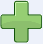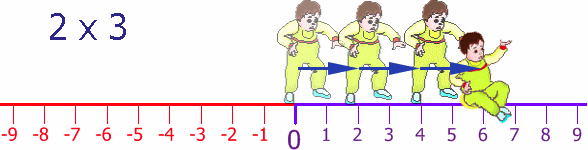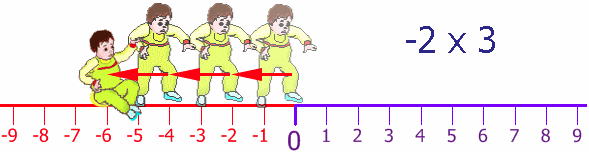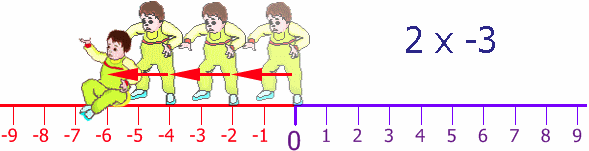# Multiplying Negatives

## When We Multiply:

 Example×two positives make a positive:3 × 2 = 6×two negatives make a positive:(−3) × (−2) = 6×a negative and a positive make a negative:(−3) × 2 = −6×a positive and a negative make a negative:3 × (−2) = −6

Yes indeed, two negatives make a positive, and we will explain why, with examples!

## Signs

"+" is the positive sign, "−" is the negative sign.

When a number has no sign it usually means that it is positive.

Example: 5 is really +5

And we can put () around the numbers to avoid confusion.

Example: 3 × −2 can be written as 3 × (−2)

## Two Signs: The Rules"Two like signs make a positive sign,
two unlike signs make a negative sign"

### Example: (−2) × (+5)

The signs are and + (a negative sign and a positive sign), so they are unlike signs (they are different to each other)

So the result must be negative:

(−2) × (+5) = −10

### Example: (−4) × (−3)

The signs are and (they are both negative signs), so they are like signs (like each other)

So the result must be positive:

(−4) × (−3) = +12

## Why does multiplying two negative numbers make a positive?

Well, first there is the "common sense" explanation:When I say "Eat!" I am encouraging you to eat (positive)

But when I say "Do not eat!" I am saying the opposite (negative).

Now if I say "Do NOT not eat!", I am saying I don't want you to starve, so I am back to saying "Eat!" (positive).

So, two negatives make a positive, and if that satisfies you, then you don't need to read any more.

## Direction

It is all about direction. Remember the Number Line?Well here we have Baby Steven taking his first steps. He takes 2 paces at a time, and does this three times, so he moves 2 steps x 3 = 6 steps forward:Now, Baby Steven can also step backwards (he is a clever little guy). His Dad puts him back at the start and then Steven steps backwards 2 steps, and does this three times:Once again Steven's Dad puts him back at the start, but facing the other way. Steven takes 2 steps forward (for him!) but he is heading in the negative direction. He does this 3 times:Back at the start again (thanks Dad!), still facing in the negative direction, he tries his backwards walking, once again taking two steps at a time, and he does this three times:So, by walking backwards, while facing in the negative direction, he moves in the positive direction.

Try it yourself! Try walking forwards and backwards, then again but facing the other direction.

## More Examples

### Example: Money

 Sam gives you three \$10 notes: +3 × +10 = you gain \$30 Sam gives you three \$10 debts: +3 × −10 = you lose \$30 Sam takes three \$10 notes from you: −3 × +10 = you lose \$30 Sam takes three \$10 debts from you: −3 × −10 = you gain \$30

### Example: A Video of People Running

People Run Forward, Video Normal:

All normal, people running forwards: +1 × +1 = +1

People Run Forward, but Video in Reverse:

Looks like people running backwards: +1 × −1 = −1

People Run Backward, Video Normal:

You see people running backwards: −1 × +1 = −1

People Run Backward, but Video in Reverse:

Looks like people running forwards: −1 × −1 = +1

### Example: Tank Levels Rising/Falling

The tank has 30,000 liters, and 1,000 liters are taken out every day. What was the amount of water in the tank 3 days ago?

We know the amount of water in the tank changes by −1,000 every day, and we need to subtract that 3 times (to go back 3 days), so the change is:

−3 × −1,000 = +3,000

The full calculation is:

30,000 + (−3 × −1,000) = 30,000 + 3,000 = 33,000

So 3 days ago there were 33,000 liters of water in the tank.

## Multiplication Table

Here is another way of looking at it.

× 1 2 3 4
1 1 2 3 4
2 2 4 6 8
3 3 6 9 12
4 4 8 12 16

Now see what happens when we head into negatives!

Let's go backwards through zero:

× 1 2 3 4
-4 -4 -8 -12 -16
-3 -3 -6 -9 -12
-2 -2 -4 -6 -8
-1 -1 -2 -3 -4
0 0 0 0 0
1 1 2 3 4
2 2 4 6 8
3 3 6 9 12
4 4 8 12 16

Look at the "4" column: it goes -16, -12, -8, -4, 0, 4, 8, 12, 16. Getting 4 larger each time.

Look over that table again, make sure you are comfortable with how it works, because ...

... now we go further to the left, through zero:

× -4 -3 -2 -1 0 1 2 3 4
-4 16 12 8 4 0 -4 -8 -12 -16
-3 12 9 6 3 0 -3 -6 -9 -12
-2 8 6 4 2 0 -2 -4 -6 -8
-1 4 3 2 1 0 -1 -2 -3 -4
0 0 0 0 0 0 0 0 0 0
1 -4 -3 -2 -1 0 1 2 3 4
2 -8 -6 -4 -2 0 2 4 6 8
3 -12 -9 -6 -3 0 3 6 9 12
4 -16 -12 -8 -4 0 4 8 12 16

Same pattern: we can follow along a row (or column) and the values change consistently:

• Follow the "4" row along: it goes -16, -12, -8, -4, 0, 4, 8, 12, 16. Getting 4 larger each time.
• Follow the "-4" row along: it goes 16, 12, 8, 4, 0, -4, -8, -12, -16. Getting 4 smaller each time.
• etc...

So it all follows a neat pattern!

## What About Multiplying 3 or More Numbers Together?

Multiply two at a time and follow the rules.

### Example: What is (−2) × (−3) × (−4) ?

First multiply (−2) × (−3). Two like signs make a positive sign, so:

(−2) × (−3) = +6

Next multiply +6 × (−4). Two unlike signs make a negative sign, so:

+6 × (−4) = −24

Result: (−2) × (−3) × (−4) = −24